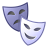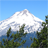# Challenging

2087
6
11-14-2011 12:19 PMNew Contributor
How can you rank the "circularity" of a polygon? Meaning that having many I wanted to calculate the most similar to a circle...
In general, how do I compare a polygon to another? I  have to assess the best site compatibility according to a building design (Comparing the area of the site, with the area of the building)
Thanks
Tags (3)
6 RepliesbyEsri Regular Contributor
In ArcGIS 10 you can use the Minimum Bounding Geometry - CIRCLE option (ArcInfo licensing) to create a bounding circle around a polygon. Then you can calculate the area ratio and the perimeter ratio between the polygon and the circle; the closer to 1 the ratios are the closer the polygon to the circle.

For the second part of your post, could you illustrate your buildings and the site polygons to help readers better understand the case? I am not sure if you have the building features already or if you are looking for sites that fit buildings of certain size and shape.New Contributor
ok Minimum Bounding Geometry, then, do you think it is correct to calculate the ratio of the bounding (circle) and the bounded polygon area?
For the second part of the thread, I have to find a site to put down a building with a list of design restrictions (geometric). So, correct the point is indentifying the site according to several constraints.byEsri Regular Contributor
The area ratio = polygonArea / minBoundingCircleArea. For example the value could be 0.7, 0.9, etc. If the polygon is close to the circle, then the area ratio will be close to 1.

The perimeter ratio = polygonShape_length / minBoundingCircleShape_length. For example the value could be 1.5, 2.8, etc. If the polygon is close to the circle, then the perimeter ratio will be close to 1. It is not enough to evaluate area only; you need to take into account both ratios.

For the second part, can you describe what the constraints are?New Contributor
The area ratio = polygonArea / minBoundingCircleArea. For example the value could be 0.7, 0.9, etc. If the polygon is close to the circle, then the area ratio will be close to 1.

The perimeter ratio = polygonShape_length / minBoundingCircleShape_length. For example the value could be 1.5, 2.8, etc. If the polygon is close to the circle, then the perimeter ratio will be close to 1. It is not enough to evaluate area only; you need to take into account both ratios.

For the second part, can you describe what the constraints are?

There are other design restrictions which deal with the proposed geometry of the site (geometry can consist of area, perimeter, width, length, etc).
What is the best site given the potential geometric restrictions?byEsri Regular Contributor
I don't have a solution or further suggestions at this point, but I am interested in knowing all the criteria in thinking of a new tool design, say Maximum Inscribing Geometry. I have seen a few similar posts on this subject. Anyone's input would be welcome!Occasional Contributor III
The metric you want is called the isoperimetric quotient.

http://mathforum.org/library/drmath/view/55406.html